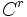# Sheaf of multiply differentiable functions

This article describes a sheaf on a manifold (the manifold may possess some additional structure in terms of which the sheaf is defined)
View other sheaves on manifolds

## Definition

Let$M$ be a$C^r$-manifold. The sheaf of$C^r$-functions' on$M$, also called the sheaf of$r$ times differentiable functions, is defined as follows:

• To each open set$U$, it associates the ring (or rather$\R$-algebra) of$C^r$-functions on$U$
• The restriction is the usual function restriction

The sheaf of$C^r$-functions in fact completely encodes the$C^r$-structure. In other words, given a topological manifold with a sheaf of functions that is supposed to be the sheaf of$C^r$-functions, the$C^r$-structure on the manifold is dictated by the sheaf.

## Facts

### Étale space is non-Hausdorff

The étale space of the shead of multiply differentiable functions is not Hausdorff. This is essentially because there can be$C^r$-functions which look the same in one direction but different in others.

The Hausdorffization of the étale space yields the space of polynomial functions of degree at most$r$.

Further information: Hausdorffization of étale space of multiply differentiable functions